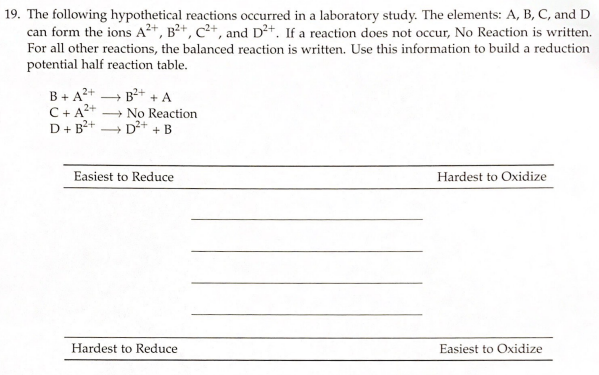# 19. The following hypothetical reactions occurred in a laboratory study. The elements: A, B, C, and D can form the ions A^2+, B^2+, C^2+, and D^2+. If a reaction does not occur, No Reaction is written. For all other reactions, the balanced reaction is written. Use this information to build a reduction potential half reaction table. B + A^2+ → B^2+ + A C + A^2+ → No Reaction D + B^2+ → D^2+ + B